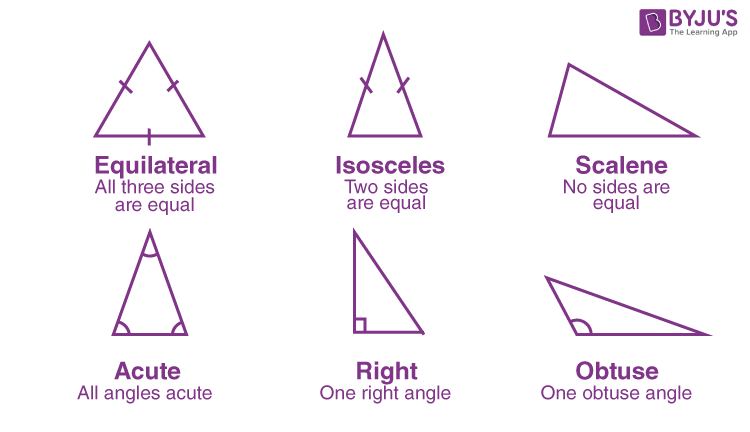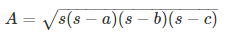# What Are Triangles?

In Geometry, a triangle is a closed two-dimensional figure with 3 sides, 3 angles and 3 vertices. Triangles are considered as a polygon with the least number of sides. In this article, let us discuss what are triangles, its types, properties, and formulas in detail. Learn triangles in detail here.

## What are Triangles in Geometry?

In Geometry, triangles are the type of polygons, which have three sides and three vertices. This is a two-dimensional figure with three straight sides. A triangle is considered a 3-sided polygon. The sum of all the three angles of a triangle is equal to 180°. The triangle is contained in a single plane. Based on its sides and angle measurement, the triangle has six types.

### How many sides does a triangle have?

A triangle has only three sides, that are connected to each other through the vertices. Therefore, no matter what the type of triangle is, it will always have three sides.

### Types of Triangle

Based on the sides of a triangle, a triangle is classified into 3 types, namely:

1. Scalene Triangle – All the sides are of different measures.
2. Isosceles Triangle – Two sides of a triangle are of the same measure and the remaining side has a different measure.
3. Equilateral Triangle – All the 3 sides of a triangle are of the same measure.

Based on the angles of a triangle, a triangle is classified into 3 types, namely:

1. Acute Angle Triangle – All the angles of a triangle is less than 90°
2. Obtuse Angle Triangle – One of the angles of a triangle is greater than 90°
3. Right Angle Triangle – One of the angles of a triangle is equal to 90°### Properties of Triangle

1. A triangle has three sides, three angles and three vertices.
2. Sum of all three interior angles of a triangle is 180°.
3. Sum of the length of two sides of the triangle is greater than its third side.
4. The area of a triangle is the half  the product of the base and the height
5. The perimeter of a triangle is the sum of all the three sides of a triangle.

### Area of Triangle

One of the properties of a triangle is its area, which is the region covered by the three-sided polygon in a plane. The formula for any triangle’s area is given by;

Area of a Triangle = ½ × Base × Height

One more formula has been introduced by a Mathematician, which is known as Heron’s Formula. This formula is used when the sides of the triangles are known to us. It is given by;Where ‘s’ is the semi-perimeter of the triangle and given by;

s = (a+b+c)/2

Where a, b and c are the measure of the three sides of the triangle.

### Perimeter of Triangle

The perimeter of a triangle is the length covered by the sides of the triangle in a plane. The formula of the perimeter is given by;

P = a + b + c## Frequently Asked Questions on What Are Triangles

### Find the perimeter of a triangle, whose sides are 3 cm, 4 cm and 6 cm.

Given, sides of the triangle are 3 cm, 4 cm and 6 cm.

Let a = 3 cm, b = 4 cm and c = 6 cm

By the perimeter formula, we know;

P = a+b+c

P = 3+4+6 = 13 cm

### Find the perimeter of an isosceles triangle whose base is 9 cm and sides are equal to 12 cm.

For an isosceles triangle, two sides are equal to each other.

Therefore, the perimeter of an isosceles triangle, P = 2xa + b

P = 2 x 12 + 9

P = 24 + 9

P = 33 cm

### Does a triangle has two sides?

A triangle has three sides not two sides.

### What are the three types of triangles based on sides?

The three sides of triangles based on sides are:
Scalene triangle
Isosceles triangle
Equilateral triangle

### What are the three types of triangles based on angles?

The three types of triangles based on angles are:
Acute triangle
Obtuse triangle
Right triangle

Test your Knowledge on What Are Triangles